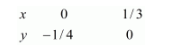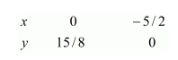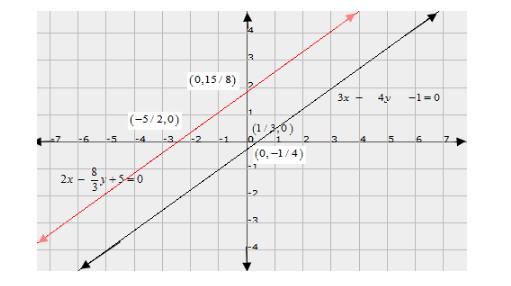# Show graphically that each one of the following systems of equations is in-consistent (i.e. has no solution) :Question:

Show graphically that each one of the following systems of equations is in-consistent (i.e. has no solution) :

$3 x-4 y-1=0$

$2 x-\frac{8}{3} y+5=0$

Solution:

The given equations are

$3 x-4 y \quad-1=0 \quad \ldots \ldots \ldots .(i)$

$2 x-\frac{8}{3} y+5=0$

$6 x-8 y+15=0 \quad \ldots \ldots \ldots .(i i)$

Putting $x=0$ in equation $(i)$, we get:

$\Rightarrow 3 \times 0-4 y=1$

$\Rightarrow y=-1 / 4$

$\Rightarrow x=0, \quad y=-1 / 4$

Putting $y=0$ in equation $(i)$ we get:

$\Rightarrow 3 x-4 \times 0=1$

$\Rightarrow x=1 / 3$

$\Rightarrow x=1 / 3, \quad y=0$

Use the following table to draw the graph.The graph of $(i)$ can be obtained by plotting the two points $A(0,-1 / 4), B(1 / 3,0)$.

$6 x-8 y=-15$...(ii)

Putting $x=0$ in equation (ii) we get:

$\Rightarrow 6 \times 0-8 y=-15$

$\Rightarrow y=15 / 8$

$\Rightarrow x=0, \quad y=15 / 8$

Putting $y=0$ in equation $(i i)$, we get:

$\Rightarrow 6 x-8 \times 0=-15$

$\Rightarrow x=-15 / 6$

$\Rightarrow x=-5 / 2, \quad y=0$

Use the following table to draw the graph.Draw the graph by plotting the two points $C(0,15 / 8), D(-5 / 2,0)$ from table.Here, the two lines are parallel.

Hence the given system of equations is inconsistent.